# Python Program to Convert Lowercase to Uppercase

This article is created to cover some programs in Python, to convert lowercase character or string to uppercase with and without using user-defined and predefined function. Here are the list of programs:

• Lowercase to Uppercase Character using ASCII Value
• Lowercase to Uppercase String using ASCII Value
• Lowercase to Uppercase using upper() Method
• Using user-defined Function
• Using Class and Object

Note - ASCII value of A is 65, B is 66, C is 67 and so on. Similarly, ASCII value of a is 97, b is 98, c is 99, and so on.

## Lowercase to Uppercase Character using ASCII Value

The question is, write a Python program to convert lowercase character entered by user to uppercase. Here is its answer. This program uses ASCII value of character to convert the character in uppercase.

```print("Enter the Character: ")
ch = input()

chup = ord(ch)
chup = chup-32
chup = chr(chup)
print("\nIts Uppercase is: ", chup)```

Here is the initial output produced by this Python program: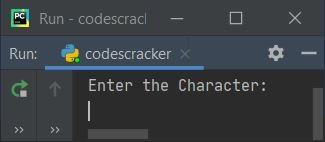Now supply the input say c as character in lowercase, then press `ENTER` key to convert and print its uppercase as shown in the snapshot given below: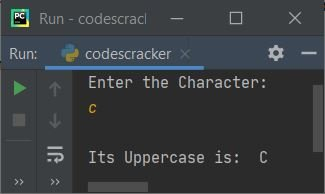Note - The ord() method is used to find Unicode value of a character passed as its argument.

Note - The chr() method is used to find the character corresponding to the Unicode value passed as its argument.

In above program, the following statement:

`chup = chup-32`

states that the value of chup gets subtracted with 32. This statement is used to initialize the ASCII value of corresponding uppercase character. For example, if entered value in lowercase is c and it gets stored in ch variable. Therefore using following statement (from above program):

`chup = ord(ch)`

the ASCII value of c, that is 99 gets initialized to chup. And using the following statement:

`chup = chup-32`

chup-32 or 99-32 or 67 gets initialized to chup. And finally, using the statement given below:

`chup = chr(chup)`

the character corresponding to the ASCII value of 67, that is C gets initialized to chup. In this way, the character gets converted into uppercase.

#### Modified Version of Previous Program

This program is the modified version of previous program, created to handle invalid input. This program uses end= to skip printing of an automatic newline using print()

```print("Enter the Character: ", end="")
ch = input()

chlen = len(ch)
if chlen==1:
if ch>='a' and ch<='z':
chup = ord(ch)
chup = chup-32
chup = chr(chup)
print("\nIts Uppercase is: ", chup)
elif ch>='A' and ch<='Z':
else:
print("\nInvalid Input!")
else:
print("\nInvalid Input!")```

Here is its sample run with user input C: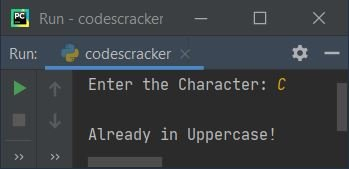Here is another sample run with user input codescracker: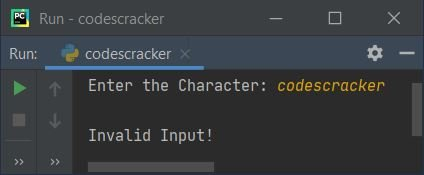## Lowercase to Uppercase String without Function

This program is created to operate with string. That is, this program converts lowercase string entered by user to its equivalent uppercase string.

```print("Enter String: ", end="")
text = input()

for i in range(len(text)):
if text[i]>='a' and text[i]<='z':
ch = text[i]
ch = ord(ch)
ch = ch-32
ch = chr(ch)
text = text[:i] + ch + text[i+1:]

print("\nIts Uppercase:", text)```

Here is its sample run with string input codescracker: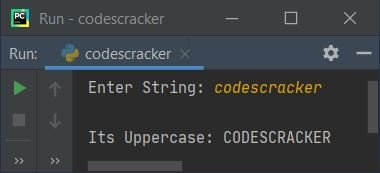In above program, I've scanned all characters of string using for loop, one by one. And compared whether the character at current index is a lowercase character or not. If the character found as a lowercase character, then that character gets converted into uppercase and using the following statement:

`text = text[:i] + ch + text[i+1:]`

new string after converting the current index's character to uppercase, gets initialized as new value of text. In above statement, text[:i] refers to all characters from 0th to (i-1)th index. And text[i+1:] refers to all characters from (i+1)th to (len-1)th index. Here len is the length of string.

For example, if text = "CODEscracker", i=4, and ch="S" therefore the following statement:

`text = text[:i] + ch + text[i+1:]`

evaluates to be, after putting the values:

`text = "CODE" + "S" + "cracker"`

states that, "CODEScracker" gets initialized to text as its new value. While slicing the string using indexing, that is [:], the index number before colon is included, whereas the index number after colon is excluded.

## Lowercase to Uppercase using upper()

This program uses upper(), a predefined method to do the same job as of previous program.

```print("Enter String: ", end="")
text = input()

text = text.upper()
print("\nIts Uppercase:", text)```

## Lowercase to Uppercase using Function

This program is created using a user-defined and a predefined method. The user-defined function used in this program is LowerToUpper(). This function returns the uppercase of string passed as its argument, using upper() method.

```def LowerToUpper(s):
return s.upper()

print("Enter String: ", end="")
text = input()

text = LowerToUpper(text)
print("\nIts Uppercase:", text)```

## Lowercase to Uppercase using Class

This is the last program of this article, created using a class, an object-oriented feature of Python.

```class CodesCracker:
def LowerToUpper(self, s):
return s.upper()

print("Enter String: ", end="")
text = input()

ob = CodesCracker()
text = ob.LowerToUpper(text)
print("\nIts Uppercase:", text)```

In above program, an object named ob is created of class CodesCracker to access its member function, LowerToUpper() using dot (.) operator.

#### Same Program in Other Languages

Python Online Test

« Previous Program Next Program »